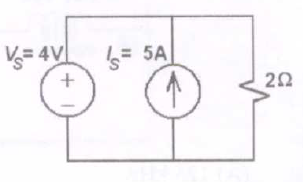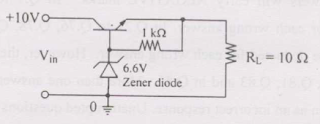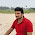## Electrical Circuits Objective Questions from Competitive Exams:

 A reactance network in the Foster's I form has poles at  ω=(infinity). The element in box-1 in the above network is  [IES2010](a) A capacitor
(b) An inductor
(c) A parallel LC circuit
(d) A series LC circuit

(e) None of the above

Statement for Linked Answer Questions 2 and 3: [GATE 2009]For the circuit given above, the Thevinin's resistance across the terminals A and B is [GATE 2009]
(a) 0.5kΩ
(c) 1kΩ
(d) 0.11kΩ
(e) None of the above

 For the circuit given above, the Thevenin's voltage across the terminals A and B is
[GATE 2009]
(a) 1.25V
(b) 0.25V
(c) 1V
(d) 0.5V
(e) None of the above
 For the circuit shown, find out the current flowing through the 2Ω resistance. Also identify the changes to be made to double the current through the 2Ω resistance.  [GATE](a) (5A; Put Vs=20V)
(b) (2A; Put Vs=8V)
(c) (5A; Put Vs=10A)
(d) (7A; Put Vs=12A)
(e) None of the above
 The common emitter forward current gain of the transistor shown is 100. The transistor is operating in [GATE 2007](a) Saturation Region
(b) Cutoff Region
(c) Reverse active region
(d) Forward active region
(e) None of the above

 The three – terminal linear voltage regulator is connected to a 10 ohm load resistor as shown in the figure. If Vin is 10V, what is the power dissipated in the transistor [GATE 2007](a) 0.6W
(b) 2.4W
(c) 4.2W
(d) 5.4W
(e) None of the above

LINKED ANSWER QUESTIONS: Q.7 to Q.8

Carry Two Marks Each Statement for Linked Answer Questions:7 & 8

In the circuit shown, the three voltmeter readings are V1=220V, V2=122V, V3=136VThe power factor of the load is  [GATE2012]
(a) 0.45
(b) 0.50
(c) 0.55
(d) 0.60
(e) None of the above

  If RL=5Ω, the approximate power consumption in the load is  [GATE2012]
(a) 700W
(b) 750W
(c) 800W
(d) 850W
(e) None of the above
Common Data Questions: 9&10
With 10V dc connected at port A in the linear nonreciprocal two-port network shown below,following were observed:
(1)1Ω connected at port B draws a current of 3A
(2) 2.5Ω connected at port B draws a current of 2AFor the same network, with 6V dc connected at port A, 1Ω connected at port B draws 7/3 A. If 8V dc is connected to port A, the open circuit voltage at port B is  [GATE2012]
(a) 6V
(b) 7V
(c) 8V
(d) 9V
(e) None of the above

  With 10V dc connected at port A, the current drawn 7Ω connected at port B is  [GATE2012]
(a) 3/7A
(b) 5/7A
(c) 1A
(d) 9/7A
(e) None of the above

5:59 PM

#### 3 comments:

1.Qn No.2, Answer is 0.2Kohms

2.For maximum depth, use lower frequencies.

3.For maximum depth, use reduced frequencies.